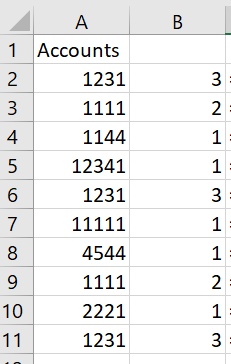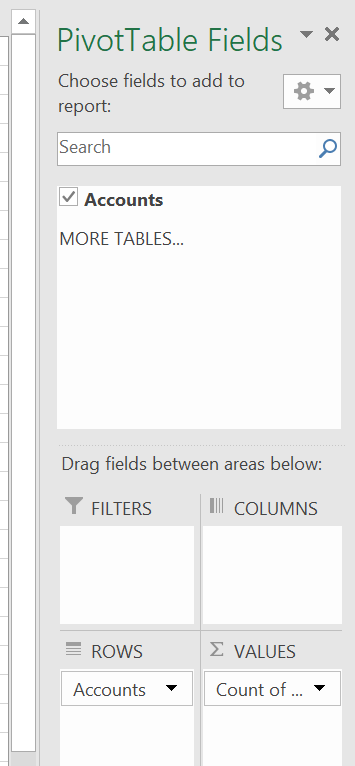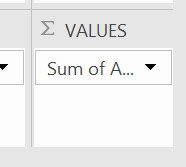# Find and Highlight Duplicates in Excel

You can quickly find duplicates in Excel by employing a few different strategies. Often users want to see if there is a duplicate email in a list. Also, retailers may want to see if there have been duplicate transactions over a given period of time. There are tons of scenarios where you may want to count duplicates. Learn Excel Beginner to Excel with simple course.

## Conditional Formatting  – Highlight Duplicates

The first way to identify duplicate values is to simply use the tools that you have that are native to Excel. You can use the conditional formatting feature to find duplicate values. Follow the instructions below to see how to quickly find duplicates

1. Highlight the entire section that you want to find duplicates. This is usually a column or an array.
2. Click Conditional Formatting from the Home Tab
3. Choose Highlight Rules
4. Chose Duplicate ValuesThe result will be that it highlights all the existing duplicates with conditional formatting.## COUNTIF Function

The COUNTIF function does exactly what it says. It counts the values in cells if a condition is met. So in this case, we want to count instances where a value is duplicated. Let’s take a look a the elements of the COUNTIF functions.  COUNT( range, criteria). The range is the column or the length of data where your duplicates live and your criteria is what it use to decide when to count.  Follow the instruction below to use the COUNTIF function to find duplicates. Here is the full function. But follow the breakdown below to see this in detail.

COUNTIF(Range, Criteria)

AKA

COUNTIF(Where the duplicates are, the value you want to check)

1. Write the COUNTIF function next to the first value in your data range=COUNTIF
2. Enter the range part of the formula =COUNTIF(A2:A11
3. Enter the second part of the formula which is what do you want to evaluate in this case we want to see if the criteria equal the first cell in our data=COUNTIF(A2:A11,A2).
4. Once you have this, you need to lock the range to ensure it doesn’t change. You can do this simply by pressing F4. So the final formula will look like this: COUNTIF(\$A\$2:\$A\$11,A2).
5. Copy the formula down and it will show you how many times a particular value is duplicated.Take a look at the function at each row to see how it works. You can see that we have locked the range and the only thing that changes is the row number of the cell in the criteria section.## PIVOT TABLE

A pivot table allows you to squash your data into a summary to evaluate counts, averages, and sums. We can use a pivot table to quickly find duplicates by counting each instance a value appears. Let’s get started. You can simply click into any part of your data to create a pivot table. Let’s follow the instructions below:

1. Click into your data and choose Insert Pivot Table for the Insert Tab, The Pivot Table Fields window will appear.
2. Drag the value you want to evaluate into the ROWS section of the pivot table.
3. Drag the same value you want to in the VALUES.
4. The default is to sum the values however we want to count. Simply click the down carrot in the Value Field Settings and choose count option.We still need to change the aggregation to count because this is not the default.Click the downward arrow or carrot to change the aggregation from SUM to COUNT.The final table will look like this.#### Gaelim Holland

Subscribe
Notify of